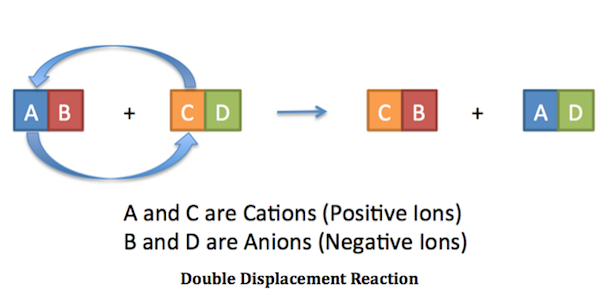# Question #4cc71

Sep 12, 2017

Double displacement reaction is a chemical reaction in which 2 ionic compound change switch their components to make new compound, you can think about is as swapping of anions or cations between the two compounds:Let us take for example:

$B a C {l}_{2} + N {a}_{2} S {O}_{4} \rightarrow B a S {O}_{4} + 2 N a C l$

$B {a}^{2 +} 2 C {l}^{-} + 2 N {a}^{+} S {O}_{4}^{2 -}$

by switching the anions or cations we get:
$B {a}^{2 +} S {O}_{4}^{2 -} + 2 N {a}^{+} 2 C {l}^{-}$

$B {a}^{2 +} 2 C {l}^{-} + 2 N {a}^{+} S {O}_{4}^{2 -} \rightarrow B {a}^{2 +} S {O}_{4}^{2 -} + 2 N {a}^{+} 2 C {l}^{-}$
$\rightarrow$
$B a C {l}_{2} + N {a}_{2} S {O}_{4} \rightarrow B a S {O}_{4} + 2 N a C l$